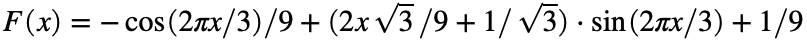# Problem 52467. Easy Sequences 2: Trigonometric function with integral input and output

The function 'F', defined as:,
will always return an integer if the input is a natural number (in radians). Furthermore, since the cosine and sine of 0, are 1 and 0 respectively, therefore F(0) = 0. For a given natural number 'n' your task is to find the value of F(n).

### Solution Stats

17.19% Correct | 82.81% Incorrect
Last Solution submitted on Nov 18, 2023

### Community Treasure Hunt

Find the treasures in MATLAB Central and discover how the community can help you!

Start Hunting!